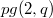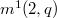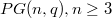﻿

## Algebraic curves, arcs, and caps over finite fields - 1986

 J. W. P. Hirschfeld Full Version (PDF)
(Quaderni di Matematica, 5 / 1986)These notes give an account of a series of lectures at the University of Lecce as well as two at the University of Bari, all during Apri1 1986.
§§1-15 are based on the thesis , of J.-F.Voloch, a part from some background remarks and classical interpolations. They deal with the number of points on an algebraic curve over a finite field. The main results of the thesis are also contained in , §16 records some classical results on elliptic curves and §17, following Voloch , proves the existence of complete k-arcs form any values of k by taking half the points on anelliptic curve. §§18-19 discusses the values of n(2,q), the size of the smallest k- art in PG(2,q), and m'(2,q), the size of the second largest complete k- art in PC(2,q), the main result of §19 follows a proof of Segre using an improved bound for the number of points on a curve from §§ll and 14. Finally, §20 summarizes the best, known estjmates for \$m_2(d,q)\$, the irrgest size of k- cap in PG(d,q).

 Introduction PDF

 The maximum number of points on an algebraic curve PDF J. W. P. Hirschfeld 1-1

 The deduction of Serre's and Ihara's results from the Riemann hypothesis PDF J. W. P. Hirschfeld 1-4

 The essential idea in a particular case PDF J. W. P. Hirschfeld 4-7

 Weierstrass points in characteristic zero PDF J. W. P. Hirschfeld 7-8

 Fundamental definitions in algebraic geometry PDF J. W. P. Hirschfeld 8-11

 The canonical series PDF J. W. P. Hirschfeld 10-11

 The osculating hyperplane of a curve PDF J. W. P. Hirschfeld 11-14

 The generalized Wronskian PDF J. W. P. Hirschfeld 15-17

 Construction of some linear systems PDF J. W. P. Hirschfeld 18-19

 The essential construction PDF J. W. P. Hirschfeld 19-24

 Elliptic curves PDF J. W. P. Hirschfeld 24-25

 Hyperelliptic curves PDF J. W. P. Hirschfeld 25-26

 Plane curves PDF J. W. P. Hirschfeld 26-28

 The maximum number of points on an algebraic curve PDF J. W. P. Hirschfeld 28-29

 Elliptic curves: fundamental aspects PDF J. W. P. Hirschfeld 30-35

 k-arcs on elliptic curves PDF J. W. P. Hirschfeld

 k-arcs in$pg (2,q)$ PDF J. W. P. Hirschfeld 42-43

 An improvement on the bound for$m^1(2,q)$ when q is prime PDF J. W. P. Hirschfeld 44-46

 k-caps in$PG (n,q), n\geq 3$ PDF J. W. P. Hirschfeld 46-47

 Bibliography PDF J. W. P. Hirschfeld 48-49

Questo sito utilizza un cookie tecnico per consentire la corretta navigazione. Se vuoi saperne di più consulta l'informativa estesa.